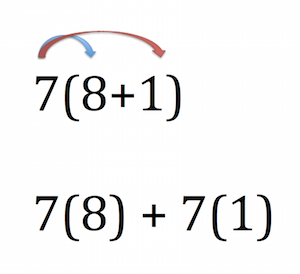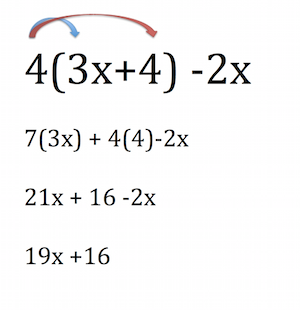# Distributive Property Lesson Plan

Instructor: Julie Zundel

Julie has taught high school Zoology, Biology, Physical Science and Chem Tech. She has a Bachelor of Science in Biology and a Master of Education.

Use Study.com's video lesson on the distributive property to introduce the concept and lead an interactive discussion on the topic. By the end of the lesson, students should now how this basic mathematical concept can help them with money management.

## Learning Objectives

After this lesson, students will be able to:

• Solve problems with variables using the distributive property
• Solve mental math problems using the distributive property
• Simplify geometry problems using the distributive property

## Length

50-65 minutes, depending on grade level and prior knowledge

## Materials

• White boards with dry erase markers or paper and pencils

## Curriculum Standards

• 6.EE Expressions and Equations

Apply the properties of operations to generate equivalent expressions.

• Expressions and Equations 8.EE

Analyze and solve linear equations and pairs of simultaneous linear equations

• 3.0A Operations and Algebraic Thinking

Understand properties of multiplication and the relationship between multiplication and division

• 3.MD Measurement and Data

Geometric measurement: understand concepts of area and relate area to multiplication and to addition

• 6.NS The Number System

Compute fluently with multi-digit numbers and find common factors and multiples

• 7.NS The Number System

Apply and extend previous understandings of operations with fractions to add, subtract, multiply, and divide rational numbers

## Instructions

• Put the following problem on the board: 4(2+4). Ask students to take a few moments to solve the problem on their white boards (or paper and pencil). Have students hold up their answers. Tell students there are a couple of ways to solve this problem, but today they will learn how to solve it using the distributive property.
• Play the first minute of the video Distributive Property: Definition, Use & Examples. Pause the video and have students complete the following problem using the distributive property: 7(8+1) on their dry erase boards. Have students hold up their answers to check for understanding.
• Complete the problem on the board to reinforce the idea. It may be worth taking a moment to place arrows (see image A) and explain that the 7 is distributed to the 8 and 1.• Continue the video, Distributive Property: Definition, Use & Examples and stop it at 1:32.
• In table groups of 2, pose the question: If you are doing mental math, why would \$6 - \$0.15 be easier to deal with than \$5.85? Before they begin, assign each student a job. Have one be the speaker (who will share the group's answer to the class) and one student be the recorder (who will write down the group's ideas).
• Give the groups 3-5 minutes, and then have each speaker share the group's ideas. Write the ideas on the classroom board (but do not tell the class if the ideas are correct or incorrect).
• Continue the video, Distributive Property: Definition, Use & Examples. Stop at 1:53 and go over that answer on the board. Tell students that the video used 6-0.15 to represent the sandwich cost. Since there were 3 sandwiches, you can figure out if you have enough money by using the distributive property.

1. 3(6-0.15) becomes 3(6) - 3(0.15)

2. Solve by multiplying and subtracting: 18-0.45=\$17.55

• Now go back to the board of their ideas and say, 'Is it easier to use 6-0.15 or 5.85 to complete the problem?' The general idea should be it is easier to multiply 3 x 6 and 3 x 0.15 compared to 3 x 5.85.
• Have students use mental math and the distributive property to figure out how much money they would need if they wanted to buy 5 burritos that cost \$4.75 each. Give them a few minutes and then have them hold up their answers to check for understanding. Take a moment to go over the problem.

1. Change \$4.90 into something easier to deal with: \$5 - \$0.10 (if they are stuck, remind them why it is simpler to use this--i.e. it is easier to multiply by .10 than .90)

2. Now set up the problem 5(5-0.10)

3. Use the distributive property: 5(5) - 5(0.10)

4. Solve: 25-0.50, so \$24.50

• Continue the video Distributive Property: Definition, Use & Examples and stop at 2:24. Have students complete the following problem using white boards: 4(3x + 4) - 2 x
• Give them a few minutes and have them show their answers. Then, depending on how well they did, go over the problem, showing something like this (image B).• Continue the video. Stop at 3:45 and have students complete the following:

(3x +4)(x-2)

• Take a moment to see how well they did, and then go over the problem using arrows (as was done in image A and B).
• Continue the video until 4:19 and then have them practice this problem:

4(2x-7) - 7(-3x + 9)

The length of a rectangle is 2 more units than the width. The total area of the rectangle is 15 square units. What is the width and length.

• Have them show their answers to check for understanding and then go over the problem.

1. Write the formula: x(x+2)=15

2. Use the distributive property to get x^2 +2x= 15.

3. Rewrite as a quadratic: x^2 + 2x - 15= 0

4. Factor: (x-3)(x+5) = 0

• If students are unfamiliar with factoring, see the video about factoring in related lessons section

5. Possible solutions for x are 3 and -5, but can't be negative so x=3

6. So the length is 5 and the width is 3

• Continue Distributive Property: Definition, Use & Examples and go until the end. As a final problem, have students do the converse of the distributive property to the following x^2 - 40x
• Give students 1-2 minutes and have them show their answer. If they take the x out they can get: x(x-40)

## Extensions

• Give students a homework assignment that covers the material presented in the video.
• You can reinforce the lesson by playing a game. Divide the class into groups of four. Each student will need a white board. On the large classroom board, start each group with 100 points (you can name the groups). Before the students get the question, each group needs to determine how much they would like to wager (groups cannot wager all of their points). Then ask a question related to the lesson. Each member of the group needs to work it out on the white board and then they need to come up with a consensus. Walk around and see what each group's answer is for the problem. If the group gets the answer correct, the points are added to their starting score and if it is incorrect the points are deducted. Continue for a several problems.

## Related Lessons

Create an account to start this course today
Try it risk-free for 30 days!Filtered by:       Clear All Filters